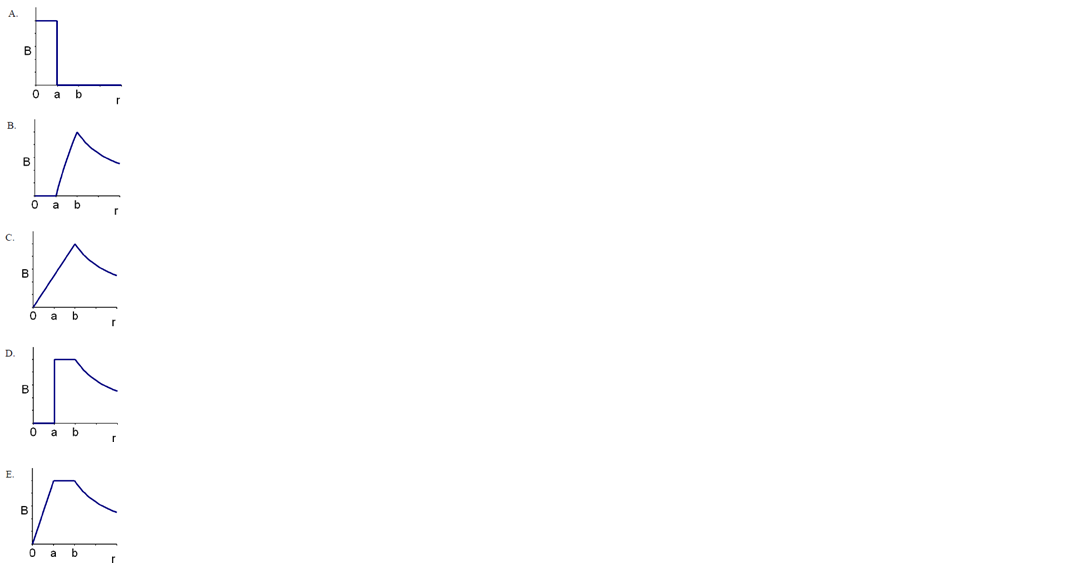# Problem: A hollow cylindrical conductor (inner radius = a, outer radius = b) carries a current I uniformly spread over its cross section. Which graph below correctly gives the magnetic field as a function of the distance r from the center of the cylinder?

###### FREE Expert Solution
90% (457 ratings)
###### Problem Details

A hollow cylindrical conductor (inner radius = a, outer radius = b) carries a current I uniformly spread over its cross section. Which graph below correctly gives the magnetic field as a function of the distance r from the center of the cylinder?